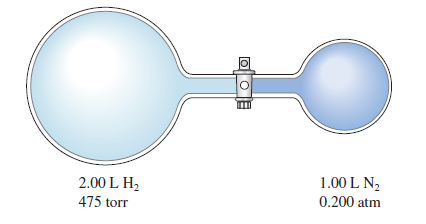Problem: Consider the flask apparatus below, which now contains 2.00 L H  2 at a pressure of 360. torr and 1.00 L N2 at an unknown pressure. If the total pressure in the flasks is 320. torr after the stopcock is opened, determine the initial pressure of N2 in the 1.00-L flask.

FREE Expert Solution
88% (435 ratings)
FREE Expert Solution

We are being asked to determine the initial pressure of N2 in the 1.00-L flask.

Recall that the ideal gas law is:

$\overline{){\mathbf{PV}}{\mathbf{=}}{\mathbf{nRT}}}$

The pressure and volume of a gas are related to the number of moles, gas constant and temperatureThe value nRT is constant

88% (435 ratings)
Problem Details

Consider the flask apparatus below, which now contains 2.00 L H  2 at a pressure of 360. torr and 1.00 L N2 at an unknown pressure. If the total pressure in the flasks is 320. torr after the stopcock is opened, determine the initial pressure of N2 in the 1.00-L flask.What scientific concept do you need to know in order to solve this problem?

Our tutors have indicated that to solve this problem you will need to apply the The Ideal Gas Law Derivations concept. You can view video lessons to learn The Ideal Gas Law Derivations Or if you need more The Ideal Gas Law Derivations practice, you can also practice The Ideal Gas Law Derivations practice problems .

How long does this problem take to solve?

Our expert Chemistry tutor, Alisa took 1 minute to solve this problem. You can follow their steps in the video explanation above.

What professor is this problem relevant for?

Based on our data, we think this problem is relevant for Professor Captain's class at UM.

What textbook is this problem found in?

Our data indicates that this problem or a close variation was asked in . You can also practice practice problems .